Anzeige

# Matrix

23. Feb 2018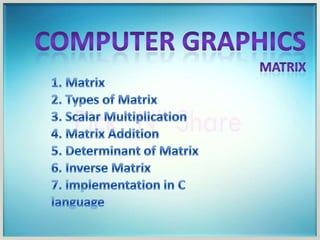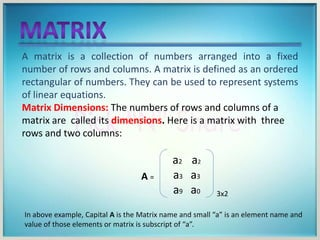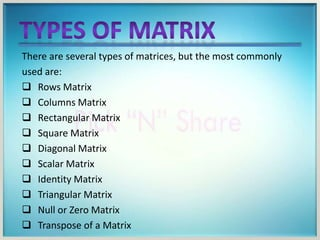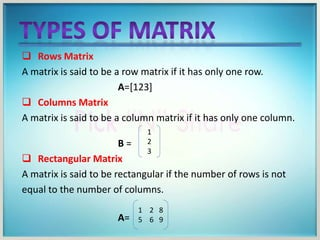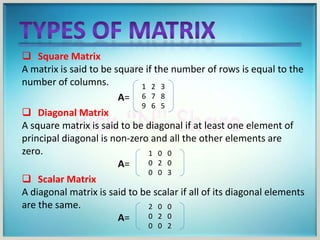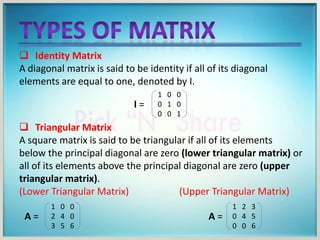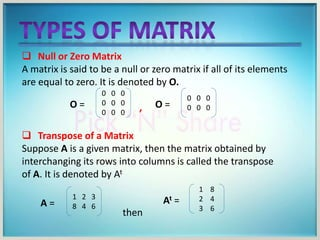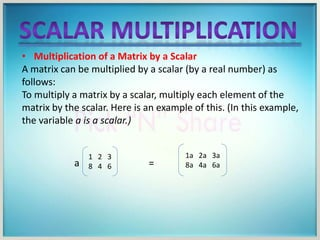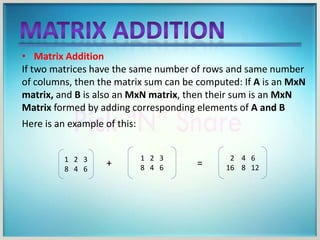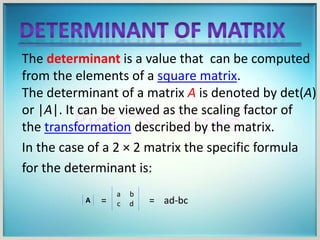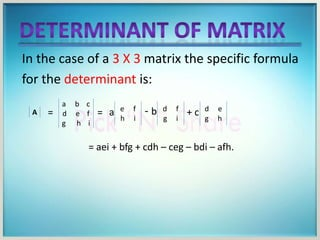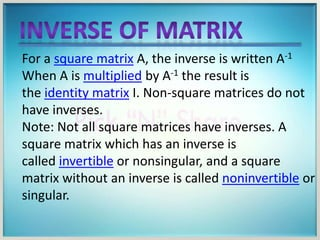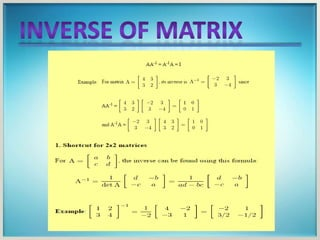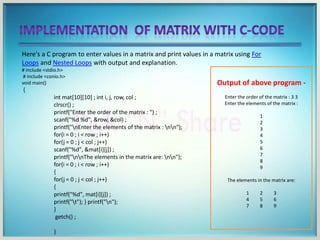1 von 14
Anzeige

### Matrix

1. A matrix is a collection of numbers arranged into a fixed number of rows and columns. A matrix is defined as an ordered rectangular of numbers. They can be used to represent systems of linear equations. Matrix Dimensions: The numbers of rows and columns of a matrix are called its dimensions. Here is a matrix with three rows and two columns: a2 a2 a3 a3 a9 a0 A = 3x2 In above example, Capital A is the Matrix name and small “a” is an element name and value of those elements or matrix is subscript of “a”.
2. There are several types of matrices, but the most commonly used are:  Rows Matrix  Columns Matrix  Rectangular Matrix  Square Matrix  Diagonal Matrix  Scalar Matrix  Identity Matrix  Triangular Matrix  Null or Zero Matrix  Transpose of a Matrix
3.  Rows Matrix A matrix is said to be a row matrix if it has only one row. A=  Columns Matrix A matrix is said to be a column matrix if it has only one column. B =  Rectangular Matrix A matrix is said to be rectangular if the number of rows is not equal to the number of columns. A= 1 2 3 1 2 8 5 6 9
4.  Square Matrix A matrix is said to be square if the number of rows is equal to the number of columns. A=  Diagonal Matrix A square matrix is said to be diagonal if at least one element of principal diagonal is non-zero and all the other elements are zero. A=  Scalar Matrix A diagonal matrix is said to be scalar if all of its diagonal elements are the same. A= 1 2 3 6 7 8 9 6 5 1 0 0 0 2 0 0 0 3 2 0 0 0 2 0 0 0 2
5.  Identity Matrix A diagonal matrix is said to be identity if all of its diagonal elements are equal to one, denoted by I.  Triangular Matrix A square matrix is said to be triangular if all of its elements below the principal diagonal are zero (lower triangular matrix) or all of its elements above the principal diagonal are zero (upper triangular matrix). (Lower Triangular Matrix) (Upper Triangular Matrix) 1 0 0 0 1 0 0 0 1 I = A = 1 0 0 2 4 0 3 5 6 1 2 3 0 4 5 0 0 6 A =
6.  Null or Zero Matrix A matrix is said to be a null or zero matrix if all of its elements are equal to zero. It is denoted by O. ,  Transpose of a Matrix Suppose A is a given matrix, then the matrix obtained by interchanging its rows into columns is called the transpose of A. It is denoted by At then 0 0 0 0 0 0 0 0 0 O = 0 0 0 0 0 0O = 1 2 3 8 4 6A = 1 8 2 4 3 6 At =
7. • Multiplication of a Matrix by a Scalar A matrix can be multiplied by a scalar (by a real number) as follows: To multiply a matrix by a scalar, multiply each element of the matrix by the scalar. Here is an example of this. (In this example, the variable a is a scalar.) 1 2 3 8 4 6a = 1a 2a 3a 8a 4a 6a
8. • Matrix Addition If two matrices have the same number of rows and same number of columns, then the matrix sum can be computed: If A is an MxN matrix, and B is also an MxN matrix, then their sum is an MxN Matrix formed by adding corresponding elements of A and B Here is an example of this: 1 2 3 8 4 6 + 1 2 3 8 4 6 = 2 4 6 16 8 12
9. The determinant is a value that can be computed from the elements of a square matrix. The determinant of a matrix A is denoted by det(A) or |A|. It can be viewed as the scaling factor of the transformation described by the matrix. In the case of a 2 × 2 matrix the specific formula for the determinant is: A = a b c d = ad-bc
10. In the case of a 3 X 3 matrix the specific formula for the determinant is: A = a b c d e f g h i = a e f h i - b d f g i + c d e g h = aei + bfg + cdh – ceg – bdi – afh.
11. For a square matrix A, the inverse is written A-1 When A is multiplied by A-1 the result is the identity matrix I. Non-square matrices do not have inverses. Note: Not all square matrices have inverses. A square matrix which has an inverse is called invertible or nonsingular, and a square matrix without an inverse is called noninvertible or singular.
12. Here's a C program to enter values in a matrix and print values in a matrix using For Loops and Nested Loops with output and explanation. # include <stdio.h> # include <conio.h> void main() { int mat ; int i, j, row, col ; clrscr() ; printf("Enter the order of the matrix : ") ; scanf("%d %d", &row, &col) ; printf("nEnter the elements of the matrix : nn"); for(i = 0 ; i < row ; i++) for(j = 0 ; j < col ; j++) scanf("%d", &mat[i][j]) ; printf("nnThe elements in the matrix are: nn"); for(i = 0 ; i < row ; i++) { for(j = 0 ; j < col ; j++) { printf("%d", mat[i][j]) ; printf("t"); } printf("n"); } getch() ; } Output of above program - Enter the order of the matrix : 3 3 Enter the elements of the matrix : 1 2 3 4 5 6 7 8 9 The elements in the matrix are: 1 2 3 4 5 6 7 8 9
Anzeige